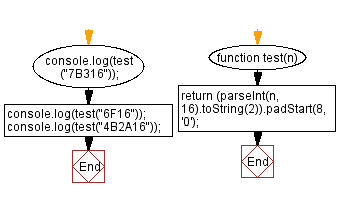# JavaScript: Hexadecimal number to binary equivalent

## JavaScript Math: Exercise-74 with Solution

Write a JavaScript program to convert a hexadecimal number to its binary equivalent (as a string).

From Wikipedia –
In mathematics and computing, the hexadecimal (also base-16 or simply hex) numeral system is a positional numeral system that represents numbers using a radix (base) of 16. Unlike the decimal system representing numbers using 10 symbols, hexadecimal uses 16 distinct symbols, most often the symbols "0"–"9" to represent values 0 to 9, and "A"–"F" (or alternatively "a"–"f") to represent values from 10 to 15.
A binary number is a number expressed in the base-2 numeral system or binary numeral system, a method of mathematical expression which uses only two symbols: typically "0" (zero) and "1" (one).

Test Data:
("7B316") -> "1111011001100010110"
("6F16") -> "110111100010110"
("4B2A16") -> "10010110010101000010110"

Sample Solution:

HTML Code:

``````<!DOCTYPE html>
<html>
<meta charset="utf-8">
<title>JavaScript program to Hexadecimal number to binary equivalent</title>
<body>

</body>
</html>
```
```

JavaScript Code:

``````function test(n){
}
console.log(test("7B316"));
console.log(test("6F16"));
console.log(test("4B2A16"));
```
```

Sample Output:

```1111011001100010110
110111100010110
10010110010101000010110
```

Flowchart:Live Demo:

See the Pen javascript-math-exercise-74 by w3resource (@w3resource) on CodePen.

Improve this sample solution and post your code through Disqus

What is the difficulty level of this exercise?

Test your Programming skills with w3resource's quiz.

﻿